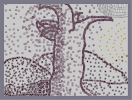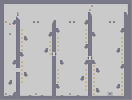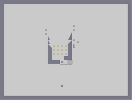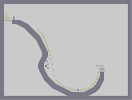### a second befor a carchrashHover over the thumbnail for a full-size version.

Author Oldan author:oldan car crash enjoy n-art rated tree 2009-11-24 2009-11-24 3 by 9 people. \$a second befor a carchrash#Oldan#enjoy#00000000000000000000000000000000000000000000000000000000000000000000000000000000000000000000000000000000000000000000000000000000000000000000000000000000000000000000000000000000000000000000000000000000000000000000000000000000000000000000000000000000000000000000000000000000000000000000000000000000000000000000000000000000000000000000000000000000000000000000000000000000000000000000000000000000000000000000000000000000000000000000000000000000000000000000000000000000000000000000000000000000000000000000000000000000000000000000000000000000000000000000000000000000000000000000000000000000000000000000000000000000000000000000000000000000000000000000000000000000000000000000000000000000000000000000000000000000000000000|3^546,572!3^539,572!3^532,569!3^527,564!3^517,557!3^515,554!3^512,552!3^506,543!3^506,542!3^505,541!3^500,537!3^498,533!3^493,527!3^490,521!3^487,514!3^484,508!3^484,503!3^484,499!3^484,493!3^484,487!3^486,481!3^488,474!3^492,468!3^496,463!3^497,460!3^498,453!3^499,445!3^500,439!3^502,430!3^502,422!3^503,416!3^503,412!3^505,408!3^505,407!3^505,404!3^505,399!3^505,394!3^502,387!3^499,381!3^498,378!3^496,372!3^496,359!3^497,352!3^498,347!3^500,339!3^501,335!3^501,331!3^502,327!3^500,324!3^498,321!3^498,314!3^498,310!3^498,304!3^500,299!3^500,297!3^502,293!3^503,290!3^503,285!3^508,280!3^509,278!3^511,275!3^511,272!3^511,270!3^511,266!3^511,264!3^510,259!3^508,255!3^507,251!3^507,248!3^507,240!3^504,231!3^502,224!3^501,220!3^502,214!3^503,211!3^506,205!3^509,196!3^511,186!3^514,180!3^520,174!3^522,170!3^525,165!3^528,160!3^530,155!3^533,150!3^536,145!3^541,141!3^546,138!3^556,133!3^560,130!3^566,127!3^570,126!3^574,125!3^580,123!3^582,121!3^586,120!3^590,120!3^596,120!3^599,119!3^606,119!3^610,119!3^615,119!3^617,119!3^624,118!3^629,117!3^634,117!3^640,119!3^645,119!3^653,119!3^660,120!3^663,120!3^668,118!3^669,116!3^666,110!3^659,108!3^653,106!3^646,104!3^639,104!3^629,104!3^628,104!3^623,103!3^618,103!3^611,103!3^606,103!3^595,103!3^588,103!3^579,103!3^573,103!3^570,103!3^560,103!3^552,103!3^543,104!3^535,105!3^530,105!3^525,108!3^521,110!3^517,111!3^513,115!3^511,115!3^506,118!3^501,120!3^497,122!3^490,123!3^480,126!3^478,127!3^473,131!3^469,134!3^345,176!3^347,174!3^349,169!3^350,163!3^350,154!3^351,147!3^351,139!3^351,135!3^351,130!3^351,120!3^351,114!3^348,109!3^348,105!3^347,97!3^344,92!3^342,84!3^339,77!3^337,74!3^335,70!3^332,66!3^326,60!3^321,57!3^317,53!3^315,51!3^308,48!3^305,46!3^304,46!3^300,44!3^294,42!3^291,42!3^288,42!3^281,42!3^272,42!3^270,44!3^258,46!3^251,47!3^249,50!3^240,52!3^235,57!3^235,54!3^238,47!3^241,35!3^244,26!3^248,23!3^253,19!3^393,23!3^395,23!3^398,24!3^399,25!3^406,26!3^408,29!3^412,29!3^413,33!3^415,36!3^419,40!3^422,44!3^426,53!3^429,55!3^433,61!3^433,71!3^434,72!3^436,74!3^437,78!3^437,82!3^437,87!3^437,89!3^437,93!3^437,97!3^437,102!3^437,105!3^437,109!3^437,111!3^437,115!3^437,123!3^437,124!3^437,129!3^439,133!3^441,138!3^443,154!3^443,156!3^443,162!3^443,172!3^442,180!3^468,131!3^465,134!3^457,137!3^453,140!3^450,145!3^344,171!3^342,171!3^338,171!3^335,166!3^327,166!3^323,165!3^316,165!3^312,166!3^307,166!3^295,167!3^289,169!3^281,173!3^274,176!3^269,182!3^267,184!3^262,189!3^260,193!3^258,196!3^253,202!3^248,208!3^246,212!3^254,212!3^259,207!3^273,203!3^279,199!3^285,196!3^290,192!3^300,190!3^310,189!3^319,189!3^323,189!3^333,189!3^342,189!3^315,159!3^311,156!3^308,152!3^299,149!3^292,146!3^285,145!3^281,144!3^272,144!3^259,144!3^251,144!3^240,144!3^241,137!3^247,130!3^250,126!3^257,123!3^263,123!3^269,123!3^277,123!3^285,122!3^291,123!3^300,123!3^307,126!3^313,126!3^319,126!3^322,126!3^336,128!3^341,133!3^350,138!3^443,99!3^445,94!3^447,91!3^454,85!3^468,81!3^473,79!3^483,78!3^492,78!3^493,78!3^482,87!3^473,90!3^472,94!3^470,100!3^466,113!3^465,120!3^465,128!3^340,192!3^338,194!3^336,196!3^335,202!3^335,207!3^334,210!3^331,212!3^331,217!3^330,223!3^330,230!3^330,234!3^330,242!3^330,246!3^330,254!3^330,261!3^334,268!3^334,272!3^331,281!3^327,286!3^325,292!3^324,300!3^324,308!3^324,315!3^324,323!3^324,331!3^324,341!3^324,347!3^323,354!3^323,358!3^321,363!3^318,371!3^318,381!3^318,389!3^323,398!3^323,407!3^324,417!3^324,424!3^325,438!3^320,441!3^317,446!3^315,453!3^313,460!3^309,463!3^306,475!3^299,491!3^300,493!3^308,469!3^307,470!3^302,477!3^300,486!3^298,499!3^298,507!3^300,513!3^300,520!3^300,529!3^301,535!3^301,545!3^301,551!3^298,558!3^296,564!3^295,569!3^324,434!3^324,422!3^324,409!3^322,390!3^319,381!3^322,365!3^497,337!3^496,346!3^496,358!3^496,367!3^503,382!3^446,166!3^446,136!3^351,173!3^355,173!3^361,175!3^363,176!3^367,180!3^340,191!3^346,199!3^349,200!3^351,206!3^446,132!3^445,140!3^444,145!3^445,161!3^449,176!3^443,177!3^438,180!3^435,183!3^309,568!3^320,567!3^331,560!3^330,549!3^317,543!3^317,525!3^320,510!3^341,498!3^371,486!3^362,463!3^354,455!3^338,462!3^319,481!3^313,497!3^358,475!3^358,512!3^356,534!3^343,527!3^316,529!3^334,534!3^353,553!3^363,561!3^379,536!3^381,517!3^386,491!3^386,464!3^379,455!3^369,435!3^359,425!3^355,400!3^353,384!3^339,403!3^342,433!3^330,443!3^320,471!3^332,510!3^346,535!3^383,545!3^406,466!3^406,418!3^386,371!3^378,342!3^362,304!3^340,354!3^359,393!3^382,424!3^388,400!3^354,341!3^355,312!3^378,271!3^386,264!3^404,321!3^406,355!3^410,386!3^412,461!3^410,493!3^399,527!3^406,556!3^421,551!3^435,512!3^435,499!3^443,457!3^445,427!3^449,392!3^460,334!3^469,309!3^488,389!3^477,427!3^472,463!3^471,520!3^484,557!3^450,570!3^449,561!3^458,513!3^423,532!3^418,541!3^391,567!3^366,568!3^365,562!3^383,544!3^363,541!3^375,507!3^340,512!3^342,516!3^353,492!3^348,469!3^337,490!3^323,471!3^330,485!3^324,498!3^327,525!3^320,549!3^356,514!3^354,479!3^357,429!3^336,434!3^337,444!3^342,440!3^345,457!3^332,466!3^335,438!3^337,452!3^358,451!3^367,464!3^334,471!3^341,479!3^325,452!3^322,478!3^314,504!3^315,546!3^340,553!3^349,550!3^357,574!3^341,565!3^347,567!3^351,564!3^364,527!3^369,518!3^360,508!3^363,492!3^373,488!3^373,462!3^378,452!3^374,445!3^374,433!3^375,421!3^375,395!3^366,399!3^348,404!3^343,409!3^345,425!3^359,434!3^374,417!3^374,406!3^355,397!3^337,406!3^338,390!3^338,376!3^332,361!3^341,333!3^341,315!3^340,301!3^349,274!3^356,271!3^362,282!3^362,297!3^369,307!3^370,316!3^370,332!3^371,347!3^373,367!3^366,372!3^361,369!3^350,356!3^349,362!3^340,357!3^337,330!3^358,327!3^362,334!3^354,301!3^341,274!3^341,294!3^351,274!3^360,275!3^349,262!3^345,250!3^353,227!3^364,246!3^371,263!3^372,281!3^383,295!3^381,326!3^382,354!3^382,365!3^382,404!3^370,288!3^377,265!3^381,251!3^385,233!3^383,219!3^383,199!3^374,242!3^359,207!3^344,201!3^326,221!3^249,217!3^325,239!3^321,240!3^315,240!3^310,240!3^305,239!3^300,237!3^293,233!3^286,229!3^283,228!3^279,226!3^271,221!3^269,218!3^251,197!3^242,191!3^238,187!3^237,183!3^234,178!3^231,171!3^229,166!3^226,161!3^223,153!3^223,141!3^223,136!3^223,127!3^227,127!3^229,130!3^254,153!3^257,156!3^264,162!3^272,168!3^359,232!3^355,249!3^356,241!3^356,229!3^371,186!3^374,168!3^372,170!3^373,174!3^370,217!3^389,194!3^387,141!3^387,125!3^386,137!3^371,147!3^368,121!3^368,148!3^368,162!3^381,181!3^373,200!3^349,220!3^364,188!3^358,225!3^374,251!3^393,270!3^393,178!3^390,167!3^392,109!3^382,84!3^369,121!3^389,100!3^372,87!3^368,76!3^370,83!3^353,71!3^369,63!3^369,35!3^370,23!3^369,28!3^365,52!3^352,53!3^361,14!3^352,49!3^351,36!3^340,35!3^336,36!3^329,37!3^318,36!3^299,26!3^289,26!3^271,30!3^260,34!3^255,40!3^275,23!3^317,51!3^333,53!3^341,48!3^350,77!3^363,95!3^372,108!3^378,118!3^377,141!3^357,154!3^375,122!3^362,98!3^368,56!3^397,61!3^409,56!3^416,111!3^413,147!3^393,81!3^409,127!3^404,146!3^418,197!3^426,224!3^416,185!3^412,225!3^407,255!3^439,312!3^433,355!3^424,345!3^428,284!3^466,259!3^451,223!3^444,249!3^480,259!3^481,208!3^494,164!3^531,135!3^500,135!3^443,151!3^459,181!3^466,177!3^476,158!3^465,225!3^476,274!3^478,304!3^475,345!3^454,371!3^484,434!3^448,480!3^460,537!3^381,423!3^415,415!3^422,383!3^405,338!3^410,313!3^427,293!3^403,261!3^414,286!3^428,182!3^419,187!3^388,91!3^394,74!3^424,132!3^419,190!3^420,245!3^426,328!3^444,369!3^434,354!3^415,406!3^412,429!3^407,506!3^422,541!3^418,563!3^403,541!3^426,481!3^424,433!3^410,393!3^397,358!3^416,290!3^314,197!3^311,208!3^305,212!3^291,206!3^308,235!3^314,210!3^298,217!3^283,216!3^255,181!3^240,159!3^238,142!3^250,185!3^243,170!3^240,162!3^259,181!3^254,157!3^220,137!3^313,207!3^307,219!3^309,224!3^319,219!3^320,211!3^329,205!3^305,204!3^299,204!3^303,224!3^325,148!3^330,151!3^339,145!3^321,133!3^302,134!3^277,131!3^261,134!3^267,142!3^308,137!3^332,156!3^329,153!3^298,130!3^341,183!3^331,182!3^324,177!3^299,176!3^288,180!3^276,190!3^270,195!3^318,175!3^306,179!3^552,117!3^454,110!3^504,270!3^441,121!10^22,527!10^25,524!10^35,519!10^41,512!10^52,508!10^58,508!10^63,507!10^69,508!10^78,512!10^86,519!10^103,524!10^111,522!10^119,522!10^132,522!10^136,519!10^141,517!10^160,511!10^164,510!10^173,509!10^184,504!10^186,502!10^195,502!10^202,499!10^208,498!10^232,489!10^248,484!10^258,484!10^265,484!10^273,484!10^282,482!10^224,496!10^218,497!10^501,481!10^512,481!10^520,481!10^525,481!10^535,482!10^543,482!10^549,482!10^556,483!10^569,483!10^581,485!10^587,485!10^605,487!10^613,488!10^622,490!10^626,490!10^631,492!10^643,498!10^650,499!10^669,500!10^674,501!10^681,501!10^689,501!10^701,501!10^708,501!10^718,503!10^726,501!10^733,497!10^741,497!10^752,497!10^657,505!10^659,499!10^588,485!10^600,484!10^764,499!10^290,483!10^242,484!10^24,380!10^32,371!10^45,364!10^61,355!10^81,352!10^108,347!10^143,353!10^161,358!10^180,365!10^219,386!10^244,402!10^203,372!10^259,410!10^278,425!10^292,442!10^239,357!10^258,343!10^280,327!10^307,321!10^522,307!10^541,313!10^572,328!10^598,351!10^618,371!10^636,398!10^663,427!10^681,463!10^689,491!10^661,384!10^698,363!10^732,342!10^761,335!0^601,245!0^644,253!0^683,259!0^725,264!0^626,211!0^668,218!0^708,223!0^671,170!0^710,188!0^748,204!0^714,141!0^745,168!0^608,299!0^649,300!0^691,301!0^625,352!0^676,344!0^713,341!0^756,333!0^674,398!0^717,383!0^755,370!0^720,430!10^664,407!10^697,421!10^709,460!10^714,482!10^754,482!10^734,454!10^754,437!10^729,413!10^706,397!10^734,365!10^540,334!10^525,368!10^529,415!10^517,451!10^543,468!10^571,468!10^602,467!10^632,472!10^663,484!10^653,457!10^635,449!10^596,449!10^558,450!10^542,443!10^546,425!10^569,425!10^602,425!10^632,429!10^611,402!10^593,400!10^566,400!10^546,398!10^544,373!10^573,377!10^592,377!10^576,357!10^560,351!10^534,351!10^514,351!10^313,342!10^288,344!10^261,353!10^253,372!10^277,388!10^297,413!10^298,386!10^276,363!10^308,367!10^106,379!10^73,381!10^52,393!10^44,408!10^37,428!10^35,448!10^38,473!10^38,488!10^53,492!10^71,489!10^74,493!10^87,499!10^110,505!10^134,506!10^149,503!10^173,493!10^184,488!10^211,477!10^221,472!10^250,471!10^267,467!10^266,454!10^256,444!10^240,436!10^227,434!10^220,425!10^212,412!10^200,403!10^279,443!10^286,468!10^246,422!10^239,417!10^210,399!10^180,373!10^165,373!10^140,370!10^126,363!10^100,363!10^76,364!10^53,375!10^35,393!10^108,412!10^74,409!10^75,394!10^61,415!10^55,431!10^61,444!10^73,466!10^96,471!10^101,482!10^121,486!10^138,486!10^168,485!10^172,478!10^193,473!10^217,463!10^234,456!10^198,439!10^162,417!10^160,397!10^134,386!10^116,386!10^93,400!10^88,440!10^112,454!10^121,465!10^133,466!10^161,466!10^172,464!10^189,452!10^152,431!10^139,433!10^121,445!10^96,434!10^81,453!10^132,413!10^185,426!10^194,393!10^158,441!10^142,451!10^185,397!10^116,430!10^84,424!10^66,469!10^44,456!10^48,431!10^79,403!10^150,424!10^173,381!10^136,412!10^188,388!10^222,423!10^252,428!10^267,427!10^279,449!10^250,418!10^231,412!10^216,398!10^206,394!10^166,385!10^138,379!10^131,378!10^81,399!10^60,415!10^54,456!10^54,480!10^92,455!10^104,399!10^154,416!10^188,459!10^217,432!10^179,414!10^195,437!10^220,450!10^258,519!10^198,530!10^158,541!10^121,551!10^70,544!10^50,541!10^60,529!10^57,522!10^46,528!10^38,547!10^31,543!10^33,533!10^34,550!10^36,570!10^27,566!10^30,554!10^34,562!10^44,561!10^41,568!10^52,572!10^59,572!10^65,573!10^75,573!10^84,572!10^95,572!10^102,571!10^113,571!10^119,570!10^126,570!10^132,570!10^138,570!10^140,568!10^144,568!10^151,568!10^160,567!10^163,567!10^172,567!10^180,567!10^186,567!10^192,567!10^196,567!10^208,567!10^214,566!10^225,566!10^236,566!10^248,566!10^265,570!10^270,571!10^244,574!10^232,574!10^215,574!10^204,574!10^190,574!10^180,574!10^149,575!10^223,575!10^251,576!10^262,573!10^275,566!10^280,567!10^280,544!10^280,539!10^284,526!10^288,505!10^288,503!10^283,508!10^280,520!10^279,538!10^281,548!10^283,555!10^287,545!10^290,536!10^284,518!10^280,505!10^280,503!10^280,493!10^283,497!10^285,494!10^285,494!10^275,494!10^265,495!10^258,495!10^248,499!10^240,502!10^234,504!10^216,517!10^204,522!10^192,524!10^192,537!10^224,506!10^235,512!10^232,509!10^247,516!10^244,524!10^254,521!10^267,508!10^258,499!10^247,505!10^250,494!10^245,494!10^241,511!10^253,524!10^261,517!10^264,530!10^258,548!10^260,553!10^266,543!10^265,552!10^265,543!10^276,523!10^275,515!10^254,506!10^245,532!10^245,542!10^235,541!10^239,542!10^246,552!10^231,553!10^223,540!10^213,549!10^197,563!10^190,553!10^205,554!10^218,554!10^237,554!10^242,556!10^260,563!10^48,539!10^57,556!10^65,555!10^49,564!10^50,552!10^58,550!10^82,561!10^61,565!10^76,555!10^100,559!10^104,564!10^123,558!10^137,558!10^149,558!10^162,557!10^171,554!10^186,549!10^206,546!10^212,537!10^214,531!10^226,529!10^210,505!10^191,512!10^185,517!10^180,527!10^178,537!10^177,555!10^170,542!10^167,525!10^180,522!10^205,516!10^230,518!10^238,525!10^148,533!10^131,535!10^120,549!10^106,540!10^90,534!10^58,520!10^90,541!10^91,545!10^104,550!10^115,531!10^138,529!10^132,540!10^87,532!10^70,533!10^108,551!10^137,538!10^154,533!10^518,498!10^506,498!10^495,498!10^500,504!10^508,520!10^520,529!10^523,535!10^532,539!10^545,557!10^556,560!10^571,564!10^551,546!10^538,543!10^524,518!10^549,515!10^534,506!10^545,506!10^580,514!10^608,524!10^601,511!10^659,520!10^662,524!10^626,529!10^582,529!10^576,552!10^623,559!10^599,564!10^588,562!10^614,541!10^677,542!10^695,544!10^655,559!10^644,570!10^715,548!10^751,532!10^728,525!10^705,524!10^727,563!10^688,566!10^740,554!10^570,520!10^561,534!10^571,499!10^600,499!10^650,513!10^529,310!10^550,318!10^568,329!10^583,334!10^595,346!10^613,352!10^631,375!10^626,372!10^642,387!10^613,357!10^629,367!10^636,374!10^661,388!10^665,392!10^640,384!10^670,401!10^684,414!10^697,417!10^716,427!10^730,434!10^734,447!10^745,467!10^758,479!10^737,440!10^731,435!10^720,427!10^711,422!10^703,419!10^693,413!10^681,406!10^672,401!10^664,392!10^650,385!10^642,380!10^578,329!10^573,328!10^563,323!10^545,316!10^535,314!10^529,312!10^520,309!10^320,320!10^311,320!10^300,321!10^289,321!10^266,327!10^259,331!10^251,336!10^242,345!10^232,353!10^220,358!10^218,364!10^25,376!10^30,375!10^32,370!10^36,365!10^42,360!10^51,358!10^60,354!10^72,351!10^81,350!10^89,350!10^94,349!10^101,346!10^107,346!10^117,345!10^132,345!10^137,346!10^150,348!10^158,353!10^166,354!10^176,355!10^183,358!10^185,363!10^199,371!10^209,372!10^214,377!10^228,383!10^231,390!10^238,395!10^244,398!10^250,401!10^257,406!10^260,411!10^270,412!10^270,415!10^277,419!10^287,422!10^291,432!10^297,436!10^299,439!10^303,448!10^515,307!10^510,307!10^585,343!10^597,346!10^605,354!10^610,356!10^765,493!10^284,325!10^733,435!10^745,449!10^747,454!10^756,464!10^762,474!3^263,279!3^175,282!3^147,264!3^107,243!3^82,176!3^125,104!3^137,167!3^86,200!3^44,220!3^45,265!3^31,305!3^38,327!3^67,296!3^57,280!3^153,295!3^321,296!3^297,299!3^271,301!3^239,303!3^220,303!3^181,308!3^326,271!3^309,268!3^284,268!3^238,276!3^224,276!3^212,272!3^313,252!3^286,253!3^262,253!3^241,253!3^231,253!3^200,254!3^266,239!3^237,241!3^217,241!3^189,238!3^168,251!3^158,261!3^247,202!3^199,212!3^189,211!3^171,217!3^165,220!3^146,202!3^139,160!3^161,195!3^193,207!3^240,219!3^221,191!3^172,176!3^156,173!3^151,163!3^192,186!3^211,188!3^159,177!3^223,217!3^234,202!3^192,144!3^196,146!3^179,152!3^172,157!3^202,151!3^179,169!3^176,305!3^211,326!3^225,331!3^284,341!3^303,351!3^303,353!3^314,336!3^280,323!3^257,328!3^231,334!3^212,334!3^206,338!3^201,345!3^201,358!3^203,374!3^202,393!3^201,415!3^202,431!3^202,451!3^205,485!3^204,505!3^201,522!3^200,542!3^213,564!3^226,567!3^248,569!3^268,569!3^235,533!3^230,510!3^232,463!3^244,529!3^254,530!3^245,461!3^273,471!3^263,416!3^271,391!3^267,350!3^253,373!3^253,430!3^256,458!3^270,434!3^282,406!3^290,387!3^291,347!3^252,342!3^258,337!3^268,328!3^279,333!3^293,291!3^296,272!3^268,293!3^265,306!3^154,280!3^225,241!3^212,65!3^193,63!3^174,68!3^164,76!3^157,87!3^148,107!3^144,128!3^145,130!3^159,121!3^199,101!3^160,116!3^201,101!3^194,104!3^184,110!3^170,120!3^190,129!3^195,111!3^203,105!3^208,99!3^213,72!3^207,88!3^183,80!3^230,81!3^246,81!3^266,66!3^293,78!3^317,92!3^320,111!3^268,103!3^264,101!3^237,111!3^219,117!3^282,86!3^311,95!3^327,95!3^299,56!3^281,56!3^229,45!3^221,38!3^226,26!3^185,44!3^179,53!3^220,41!3^207,37!3^198,49!3^168,68!3^276,376!3^229,381!3^229,368!3^284,393!3^289,403!3^298,386!3^253,419!3^337,368!3^523,96!3^501,99!3^491,98!3^485,104!3^498,110!3^473,118!3^462,71!3^454,71!3^467,84!3^461,84!3^454,89!3^478,78!3^491,78!3^476,89!3^470,106!3^469,111!1^593,24!1^593,28!1^594,30!1^601,44!1^603,47!1^604,48!1^606,52!1^606,57!1^611,58!1^615,62!1^615,65!1^620,66!1^625,66!1^628,67!1^630,68!1^631,71!1^597,29!1^598,34!1^598,38!1^604,43!1^607,49!1^613,57!1^617,60!1^624,65!1^630,74!1^632,76!1^636,77!1^639,80!1^640,81!1^644,83!1^650,86!1^653,88!1^658,90!1^660,91!1^663,92!1^668,92!1^673,92!1^677,92!1^680,94!1^686,96!1^693,97!1^698,97!1^702,97!1^706,97!1^714,97!1^718,97!1^723,97!1^726,97!1^730,96!1^734,94!1^740,94!1^749,94!1^753,91!1^759,87!1^612,28!1^613,34!1^623,38!1^623,42!1^626,45!1^631,53!1^636,57!1^641,61!1^645,62!1^648,67!1^653,70!1^658,71!1^664,73!1^668,76!1^672,76!1^677,77!1^681,80!1^688,83!1^694,83!1^709,83!1^717,83!1^725,83!1^734,82!1^746,78!1^754,75!1^762,75!1^635,33!1^640,38!1^644,39!1^645,44!1^652,47!1^653,47!1^657,50!1^669,57!1^672,58!1^678,58!1^686,64!1^694,64!1^703,64!1^714,65!1^727,65!1^742,64!1^752,63!1^760,63!1^655,29!1^667,36!1^671,36!1^676,36!1^682,38!1^685,41!1^695,44!1^704,45!1^714,45!1^728,45!1^736,45!1^752,45!1^761,46!1^698,29!1^704,34!1^720,40!1^719,36!1^720,33!1^739,35!1^750,32!1^768,32!1^690,29!1^680,26!3^84,144!3^48,108!3^60,60!3^96,60!3^84,108!3^108,180!3^36,156!3^36,192!3^96,84!3^36,24!3^296,572!3^292,572!3^292,571!3^294,567!3^295,565!3^295,561!3^295,559!3^297,555!3^298,551!3^298,549!3^298,544!3^299,541!3^299,535!3^299,531!3^299,528!3^299,524!3^299,520!3^299,516!3^299,513!3^299,509!3^299,504!3^300,500!3^300,499!3^300,496!3^300,493!3^300,489!3^300,485!3^303,482!3^303,478!3^303,475!3^307,470!3^309,465!3^309,462!3^312,457!3^313,453!3^315,449!3^317,444!3^319,441!3^321,438!3^321,434!3^322,431!3^322,426!3^322,422!3^322,417!3^322,413!3^322,409!3^322,405!3^322,401!3^322,398!3^322,394!3^322,390!3^322,384!3^322,378!3^322,376!3^322,374!3^322,373!3^322,369!3^322,366!3^322,363!3^322,360!3^322,357!3^322,355!3^322,351!3^322,349!3^322,347!3^323,345!3^323,342!3^323,338!3^323,335!3^323,330!3^323,326!3^323,322!3^323,318!3^323,315!3^323,311!3^330,307!3^324,305!3^322,292!3^323,288!3^325,280!3^325,275!3^328,271!3^328,265!3^328,258!3^328,245!3^328,243!3^325,241!3^321,240!3^313,240!3^308,240!3^305,238!3^303,237!3^299,236!3^294,235!3^290,232!3^285,228!3^279,223!3^273,221!3^267,218!3^267,218!3^257,215!3^225,134!3^224,134!3^221,136!3^220,139!3^220,144!3^220,149!3^222,153!3^223,159!3^224,159!3^225,162!3^227,165!3^231,170!3^234,178!3^238,186!3^231,129!3^233,135!3^235,136!3^239,143!3^256,147!3^258,151!3^259,156!3^263,160!3^270,164!3^263,184!3^256,190!3^253,193!3^251,197!3^250,203!3^249,214!3^248,217!3^251,216!3^262,211!3^267,206!3^271,202!3^276,198!3^282,193!3^286,190!3^293,185!3^296,184!3^300,184!3^304,184!3^308,184!3^310,184!3^317,184!3^323,184!3^334,185!3^336,163!3^332,164!3^328,164!3^321,164!3^314,163!3^305,163!3^300,163!3^294,164!3^287,165!3^284,166!3^279,169!3^272,172!3^261,175!3^261,178!3^242,157!3^247,150!3^257,148!3^261,146!3^265,144!3^269,143!3^275,143!3^279,144!3^284,144!3^297,147!3^300,148!3^304,148!3^313,151!3^319,153!3^344,136!3^339,132!3^331,131!3^324,128!3^317,123!3^309,123!3^305,123!3^300,123!3^294,123!3^288,123!3^276,123!3^270,123!3^258,124!3^251,125!3^246,135!3^245,143!3^244,147!3^244,154!3^241,162!3^350,170!3^350,169!3^350,164!3^350,162!3^351,155!3^351,146!3^351,143!3^351,136!3^351,134!3^351,130!3^351,126!3^351,119!3^351,116!3^351,109!3^351,104!3^350,98!3^349,94!3^345,87!3^338,79!3^337,77!3^335,73!3^333,67!3^329,63!3^326,59!3^321,56!3^310,52!3^299,48!3^296,46!3^292,46!3^284,44!3^279,44!3^275,44!3^270,44!3^260,42!3^257,42!3^251,44!3^245,49!3^237,48!3^237,42!3^238,37!3^240,28!3^240,26!3^248,20!3^394,20!3^400,20!3^411,24!3^412,35!3^416,39!3^419,41!3^420,47!3^423,49!3^427,51!3^428,55!3^430,66!3^430,69!3^437,83!3^439,99!3^440,120!3^440,134!3^440,144!3^440,151!3^440,160!3^440,170!3^440,175!3^437,178!3^430,180!3^426,181!3^420,185!3^456,135!3^463,133!3^467,133!3^471,131!3^466,124!3^468,114!3^469,107!3^469,97!3^469,90!3^473,84!3^479,79!3^482,77!3^465,78!3^456,82!3^450,83!3^445,89!3^481,133!3^481,131!3^488,126!3^494,123!3^495,122!3^507,119!3^509,117!3^523,112!3^528,108!3^528,106!3^535,104!3^546,104!3^550,102!3^556,102!3^563,101!3^566,101!3^572,101!3^582,102!3^589,102!3^594,102!3^596,102!3^603,101!3^606,101!3^610,101!3^617,101!3^622,101!3^627,101!3^635,101!3^636,101!3^644,103!3^657,105!3^663,106!3^668,109!3^674,119!3^670,117!3^674,119!3^676,123!3^674,124!3^668,120!3^661,120!3^658,120!3^651,120!3^645,120!3^638,120!3^630,120!3^627,120!3^622,120!3^621,121!3^618,121!3^616,121!3^610,122!3^607,122!3^597,122!3^595,121!3^591,121!3^578,121!3^576,123!3^572,126!3^569,127!3^558,129!3^556,130!3^551,133!3^547,135!3^541,137!3^537,140!3^536,144!3^531,146!3^531,150!3^530,152!3^526,154!3^522,160!3^520,163!3^520,167!3^514,169!3^510,175!3^510,178!3^508,184!3^508,193!3^508,199!3^504,203!3^503,211!3^503,217!3^503,222!3^503,230!3^507,245!3^507,240!3^507,227!3^505,217!3^506,192!3^506,199!3^507,233!3^507,241!3^507,252!3^512,268!3^510,271!3^509,277!3^507,281!3^506,284!3^505,289!3^503,293!3^503,297!3^503,301!3^502,303!3^501,307!3^500,309!3^500,313!3^500,317!3^499,323!3^500,328!3^503,332!3^503,340!3^501,344!3^497,346!3^497,348!3^497,350!3^498,360!3^497,364!3^497,370!3^497,373!3^497,377!3^498,381!3^500,382!3^503,385!3^506,396!3^504,396!3^504,400!3^504,403!3^504,410!3^504,413!3^504,417!3^503,420!3^503,423!3^502,426!3^501,432!3^499,439!3^499,444!3^499,448!3^499,457!3^498,464!3^496,468!3^490,470!3^487,472!3^487,476!3^486,481!3^486,485!3^485,489!3^485,497!3^487,507!3^487,511!3^487,519!3^490,519!3^492,524!3^493,531!3^495,534!3^498,542!3^500,545!3^503,547!3^508,555!3^520,559!3^524,561!3^533,566!3^546,NaN!3^106,320!3^93,306!3^62,318!3^83,267!3^74,249!3^28,247!3^55,237!3^68,215!3^86,222!3^123,220!0^608,224!0^657,236!0^689,241!0^651,185!0^695,202!0^728,215!0^699,152!0^726,175!0^745,129!0^678,284!0^639,278!0^598,277!0^615,331!0^661,325!0^701,324!0^744,323!0^677,379!0^718,357!0^697,431!0^727,285!0^735,301!0^741,233!0^731,247!0^752,228!10^559,161!10^537,72!10^482,54!3^565,115!3^491,232!3^471,389!3^349,338!3^345,394!3^350,434!3^343,468!3^344,413!3^354,406!3^357,417!3^360,415!3^327,416!3^335,412!3^339,408!3^340,375!3^336,358!3^340,376!3^365,382!3^377,380!3^379,386!3^354,344!3^368,359!3^368,355!3^362,353!3^330,349!3^330,343!3^340,342!3^342,326!3^348,312!3^350,311!3^345,302!3^352,295!3^351,286!3^344,272!3^348,251!3^346,239!3^343,228!3^362,220!3^375,235!3^375,253!3^375,266!3^366,255!3^364,252!3^367,218!3^369,203!3^352,189!3^356,172!3^365,135!3^368,113!3^368,88!3^380,94!3^380,98!3^347,46!3^343,58!3^358,68!3^361,50!3^350,32!3^342,20!3^328,22!3^321,32!3^312,34!3^303,33!3^281,33!3^262,33!3^112,64!3^102,42!3^35,63!3^67,94!3^58,178!3^135,318!3^110,277!3^136,44!10^538,225!10^562,271!10^607,177!10^581,71!10^575,570!10^564,568!10^527,NaN!3^540,72!3^480,48!3^456,48!3^516,36!3^540,60!3^552,96!3^540,180!3^600,162!3^531,169!3^549,197!3^582,194!3^596,137!3^556,235!3^535,277!3^561,305!3^564,47!3^552,32!3^464,32# funny and first N-Art looks like a tree or? Or...? ... Enjoy and rate and comment a faved and... please

## Other maps by this authorThe Sailor Flags Simple map Ski-jumping

Pages: (0)

agree w/ chris, too blurry though

### looks like cool

watch my maps pls

yea.

### oh no.

i hope this dont stay at the top of the hot maps page for a day or two. that gets on my nerves.